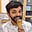RELATED TAGS

# How to do arithmetic operations in PostgreSQLMalhar Lakdawala

PostgreSQL provides many mathematical operators for common mathematical conventions. The bitwise operators can be used only with the integer data types.

Various arithmetic operations which can be done on PostgreSQL table
 Operators Description Example Output + Addition 5+8 13 - Subtraction 6-9 -3 * Multiplication 5*8 40 / Division 15/3 5 % Modulo 15%2 1 ^ Raied To 4^2 16 |/ Square Root |/16 4 ! Factorial !6 720 @ Absolute Value @-15.07 15.07

Consider the basic table below. If we want to add an integer value to the marks table, the below command can be used.

Please note, arithmetic operations can only be done on integer columns.

id student_name marks
1 Ryan 52
2 Joanna 89
3 Casey 41
4 Emmett 37
5 Andy 75

### Syntax

SELECT id, name, marks, marks + 100
AS "marks+50" FROM students;
id student_name marks marks+100
1 Ryan 52 152
2 Joanna 89 189
3 Casey 41 141
4 Emmett 37 137
5 Andy 75 175

Here, we have added 100 to each student’s marks, i.e, performed the addition operation on a single column.

Let’s perform the addition of 2 columns:

SELECT id, name, marks, marks + id
AS "marks+id" FROM students;
id student_name marks marks+id
1 Ryan 52 53
2 Joanna 89 91
3 Casey 41 44
4 Emmett 37 41
5 Andy 75 80

Let’s consider a case where we want to do a conditional arithmetic operation

Here, we are multiplying 10, whose marks are more than 50:

SELECT id, name, marks, marks +10
AS "cond_marks+10" FROM students where marks>50;
id student_name marks cond_marks+10
1 Ryan 52 62
2 Lucy 89 99
5 Robert 75 85

### Subtraction

Here, we are subtracting the marks column by 15:

SELECT id, name, marks, marks -15
AS "marks-15" FROM Students;
id student_name marks marks-15
1 Ryan 52 37
2 Lucy 89 74
3 Frank 41 26
4 Jane 37 22
5 Robert 75 60

### Multiplication

Here, we are multiplying the marks column by 12:

SELECT id, name, marks, marks *12
AS "marks*12" FROM Students;
id student_name marks marks*12
1 Ryan 52 624
2 Lucy 89 1068
3 Frank 41 492
4 Jane 37 444
5 Robert 75 900

### Division

Here, we are dividing the marks column by 3:

SELECT id, name, marks, marks /3
AS "marks/3" FROM Students;
id student_name marks marks/3
1 Ryan 52 17
2 Lucy 89 29
3 Frank 41 13
4 Jane 37 12
5 Robert 75 25

In a similar way, various arithmetic operations can be done to get the desired output.

RELATED TAGS

CONTRIBUTORMalhar Lakdawala
RELATED COURSES

View all Courses

Keep Exploring

Learn in-demand tech skills in half the time